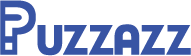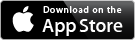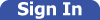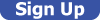## How to Solve Sudoku Puzzles

### by Roy Leban

One of the reasons that Sudoku is so popular is that it all boils down to three simple rules:

• Each row in the puzzle contains all of the numbers from 1 to 9
• Each column in the puzzle contains all of the numbers from 1 to 9
• Each 3x3 outlined box in the puzzle contains all of the numbers from 1 to 9

That’s it! Well, plus a little logic to figure it all out. There are entire books written about the logic of solving Sudoku, but learning a few simple techniques can take you a long way. For this guide, a cell is one of the 81 places in the grid to enter a single number. A box is defined as an outlined 3x3 array of cells.

#### Forced Numbers

One situation that helps determine an entry is when a row, column, or box, contains 8 of the 9 numbers. According to the basic rules, all numbers from 1 to 9 must be used so in this situation, the 9th unfilled cell must contain the remaining number.

This strategy can be expanded beyond the trivial case with only one empty cell. If there are multiple empty cells in a row, column, or box, systematically crosscheck the empty cells to see what potential candidates you can eliminate. For instance, a row has two empty cells, and you've determined that they must contain a 2 and a 4. By looking at the two crossing columns, you might see that one already contains a 2, which forces the where the 2 must go. In turn this forces the 4 to go in the last empty cell.

In an even more expanded case, let's say that a box has five empty cells and you are trying to determine where the 3 goes. By scanning across and down the rows and columns that pass through the box, you can eliminate any empty cells in a row or column that already contains a 3.

#### Groups of Three

Examining rows and columns in groups of three can be very useful. Consider three rows that all pass through the same set of three boxes. These three rows will each contain one instance of all the numbers from 1 to 9 so that each number will appear in each one of the three boxes. For example, in these three rows, a 1 will appear exactly once in the left box, once in the middle box, and once in the right box. If two of the rows have a number that appears in two of the boxes, this means that in the third row, that same number will appear somewhere in the third box.

This narrows down the options for where a given number can be placed. Since some of the cells may already be filled, you may have enough information to know where the number should actually go.

#### Taking Notes

Taking notes, or penciling in numbers, can be useful when solving. Some people find it useful to note the numbers that might be in a given cell, while other people find it useful to note the numbers that cannot be in a given cell. Whichever you do, continually update your notes as you solve so that you can narrow down the possibilities for each cell.

Suppose you pencil in all the possibilities for all the cells in a certain row. Most empty cells will have several possible numbers that could potentially fit. Other times, you will discover that there is only one possible number that can go in the cell and can thus confidently fill in the entry. Sometimes there are no cells with only a single candidate In this case, look at all the candidates for cells in a certain row, column, or box. If there is any number that appears as a candidate in only one cell in that row, column, or box, you know that it must go in that cell even though your list of candidates has other options.

If neither of these situations occur, your notes can still help. Suppose there are two cells in a row, column, or box that each have only the same two possibilities. This means that those two numbers go in those cells in some order and thus do not appear elsewhere in that row, column, or box. You can now eliminate those two numbers as possibilities in the remaining blank cells.

#### Hints

If you’re just getting started with Sudoku puzzles, don’t forget you can get hints. Just tap on the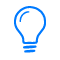icon to fill in a value or remove mistakes.

#### Sudoku Variants and Related Puzzles

There are lots of variant types of Sudoku puzzles. Many variants add additional or slightly different constraints, sometimes to make the puzzles easier and sometimes to make them harder.

Sudoku 8™ uses only the numbers 1-8 but maintains the familiar 9x9 grid to provide an easier puzzle that uses the same rules as regular Sudoku.

Three-in-a-Row Sudoku™ are easier Sudoku puzzles which add one additional rule: lines drawn in the grid indicate sequences of three consecutive numbers.

Wordoku puzzles use letters instead of the digits 1-9, with each puzzle using a different word or phrase with 9 unique letters, providing a nice variety to solving.

Check the Introduction in each ebook of variant Sudoku puzzles for more information and instructions.

Other common variants include HyperSudoku and Diagonal Sudoku (also called Sudoku X), which add additional regions containing the numbers 1-9. Symdoku puzzles use symbols instead of numbers.

There are also related puzzles which are similar to Sudoku but with different rules. For example, KenKen puzzles, sometimes called Calcudoku or Mathdoku, meet the row and column rules of Sudoku, but have cages with mathematical expressions instead of the 3x3 boxes that Sudoku puzzles have.